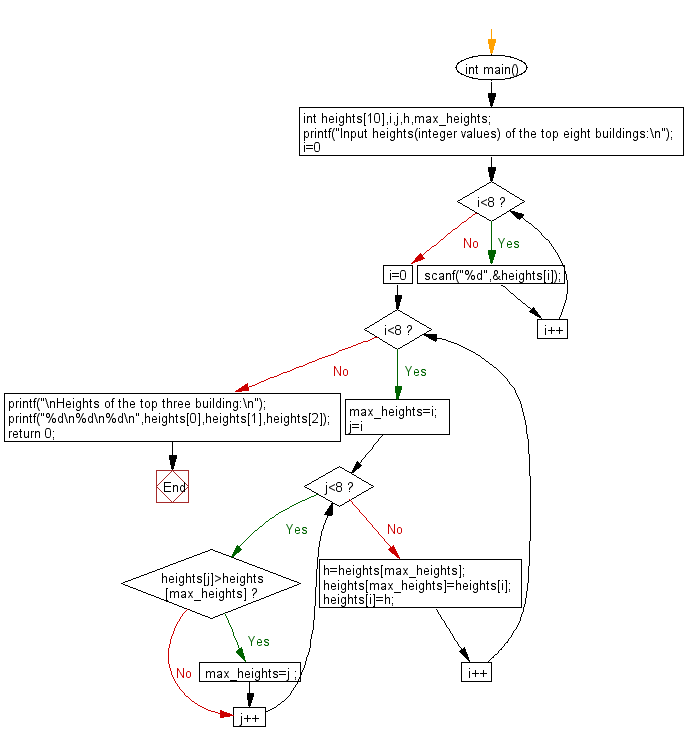﻿ C : From 8 given buildings, find the top 3 in DESC order

# C Exercises: Find heights of the top three building in descending order from eight given buildings

## C Basic Declarations and Expressions: Exercise-132 with Solution

Write a C program to find the heights of the top three buildings in descending order from eight given buildings.

Input:
0 <= height of building (integer) <= 10,000

Sample Solution:

C Code:

``````#include<stdio.h>

int main(){

int heights, i, j, h, max_heights;

// Prompt the user to input heights of the top eight buildings
printf("Input heights (integer values) of the top eight buildings:\n");

// Read the heights into the array
for(i = 0; i < 8; i++){
scanf("%d", &heights[i]);
}

// Sorting the heights in descending order
for(i = 0; i < 8; i++){
max_heights = i;
for(j = i; j < 8; j++){
if(heights[j] > heights[max_heights]){
max_heights = j;
}
}
h = heights[max_heights];
heights[max_heights] = heights[i];
heights[i] = h;
}

// Printing the heights of the top three buildings
printf("\nHeights of the top three buildings:\n");
printf("%d\n%d\n%d\n", heights, heights, heights);

return 0; // End of program
}
``````

Sample Output:

```Input heights(integer values) of the top eight buildings:
25
15
45
22
35
18
95
65

Heights of the top three building:
95
65
45
```

Flowchart:C programming Code Editor:

What is the difficulty level of this exercise?

Test your Programming skills with w3resource's quiz.

﻿